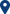# Dr. Dan Lemberg## Dr. Dan LembergM107
Department: Mechanical Engineering
Current Position: Lecturer

### Education :

#### B.Sc. 2000 – 2002, University of Haifa, Department of Mathematics 1995 – 1998, State University in Lvov, Department of Mathematics and Mechanics

Research Interests
• 1. Category theory
• 2. Set theory
• 3. Homological algebra
• 4. Data mining
• 5. Clusterization theory
• 6. Big data
• 7. Algorithmic theory

### Refereed Papers

1. V. Hinich and D. Lemberg. Non-commutative unfolding of hypersurface singularity. Journal of noncommutative geometry, 8(4):1147 – 1169, 2014. arXiv:1207.6333.
2. V. Hinich and D. Lemberg. Formality theorem and bialgebra deformations. Annales de la Faculté des sciences de Toulouse, 25(2 – 3):569 – 582, September 2016. arXiv:1410.2132.
3. D. Lemberg, A. Soffer, and Z. Volkovich. New approach for plagiarism detection.

### Teaching Experience

Programing languages,
Numerical analysis,
Algorithms in graph theory,
Compilation theory

The Technion:
Calculus I, II,
Linear algebra,
Regular differential equations,
Comlex analisys,
Modern algebra

University of Haifa:
Calculus I, II,
Linear algebra I, II,
Discrete mathematics
שינוי גודל גופנים
ניגודיות